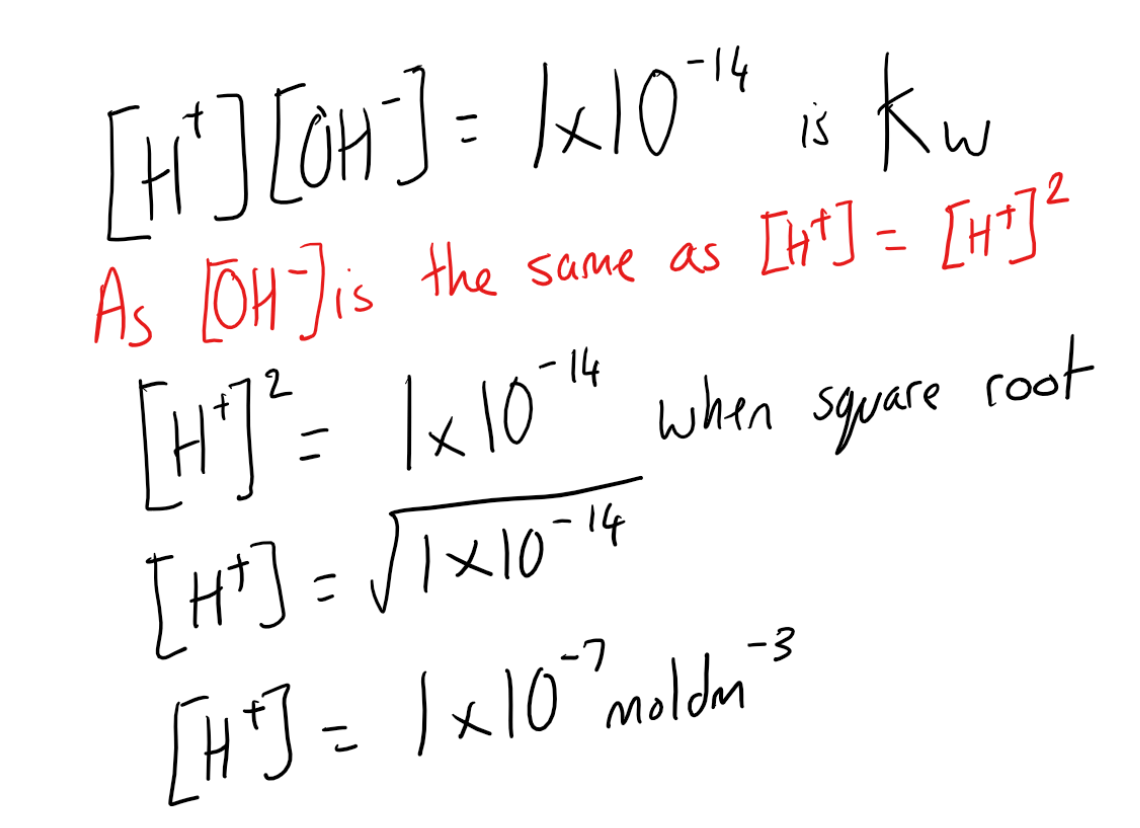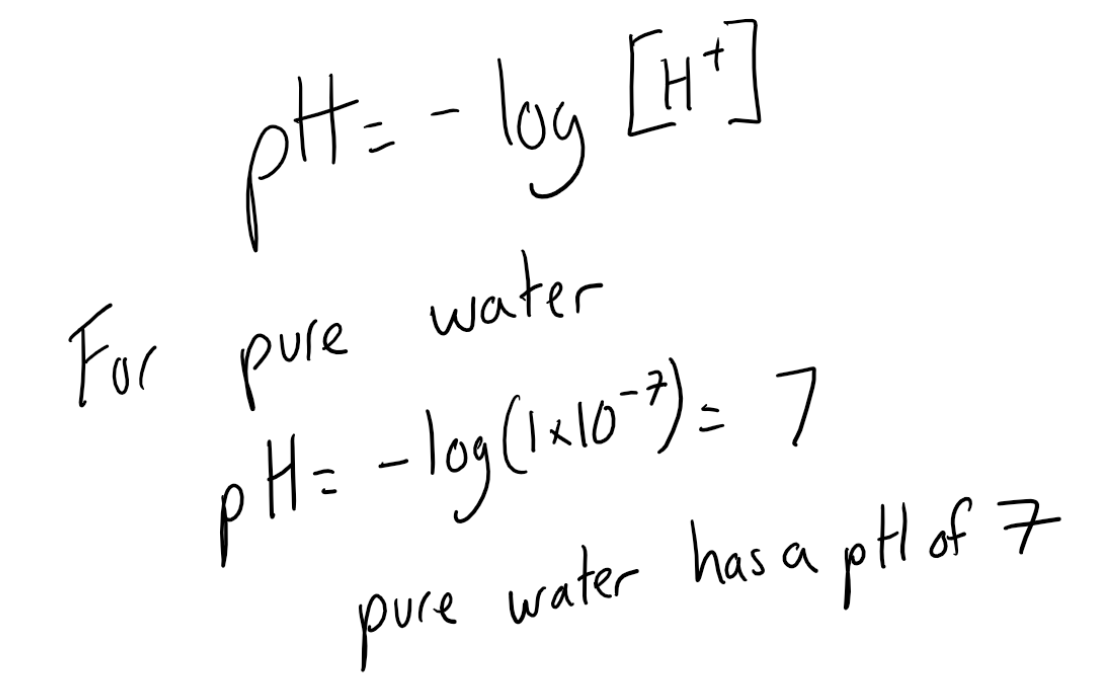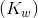Complimentary 1-hour tutoring consultation

MCAT Content / Acid Base Equilibria / Ionization Of Water

### Ionization of Water

Topic: Acid Base Equilibria

The self-ionization of water is the process in which water ionizes to hydronium ions and hydroxide ions.

The self-ionization of water (the process in which water ionizes to hydronium ions and hydroxide ions) occurs to a very limited extent. When two molecules of water collide, there can be a transfer of a hydrogen ion from one molecule to the other. The products are a positively charged hydronium ion and a negatively charged hydroxide ion.The equilibrium constant for the self-ionization of water is referred to as the ion-product for water and is given the symbol Kw and is derived from the equilibrium constant equation.The ion-product of water (Kw) is the mathematical product of the concentration of hydrogen ions and hydroxide ions. At 25°C, the experimentally determined value of  Kw in pure water is 1.0 × 10 -14 .

pH is used for indicating the concentration of H +. The letters pH stand for the power of the hydrogen ion. The pH of a solution is the negative logarithm of the hydrogen-ion concentration:

pH = -log[H+]

In pure water or a neutral solution, [H + ] = 1.0 × 10 -7  M. Substituting into the pH expression gives a value of pH 7.Practice Questions

MCAT Official Prep (AAMC)

Practice Exam 3 C/P Section Passage 6 Question 34

Practice Exam 4 C/P Section Passage 8 Question 40

Key Points

• The self-ionization of water is described and an ionization constant for the process is stated.

• Acidic and basic solutions are defined.

• Water can ionize to form a hydronium ion and hydroxide ion.

• Kw is the ionic product of water and has a value of 1×10-14.

• Using the negative logarithm of hydrogen ion concentration we can prove that pure water has a pH of 7.

• pH can be calculated by taking the -log of the H+ concentration

Key Terms

Acidic solution: A solution in which the concentration of hydrogen ions is greater than the concentration of hydroxide ions.

Basic solution: A solution in which the concentration of hydroxide ions is greater than the concentration of hydrogen ions.

Ion-product of water: The mathematical product of the concentration of hydrogen ions and hydroxide ions.

Self-ionization of water: The process in which water ionizes to hydronium ions and hydroxide ions.

pH: The negative logarithm of the hydrogen-ion concentration.

Billing Information